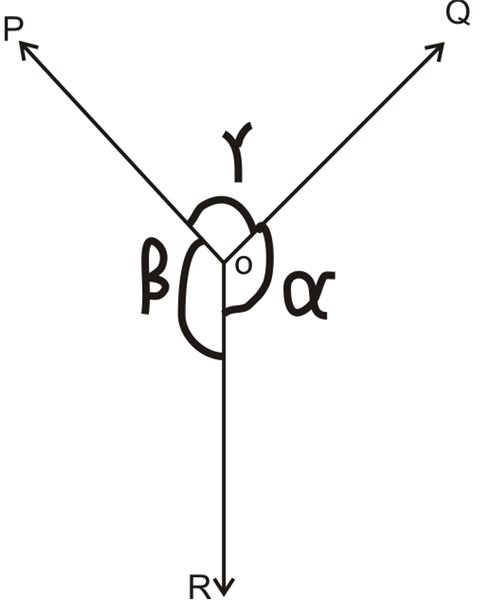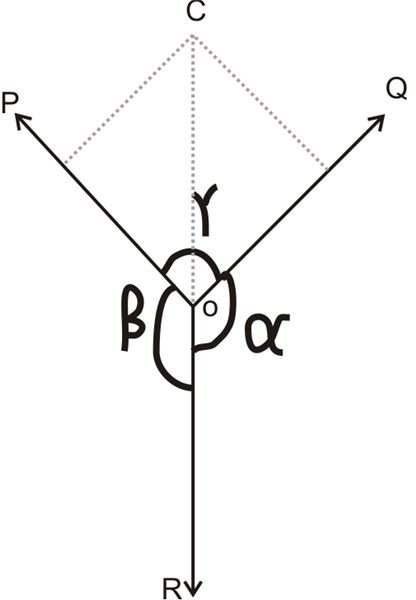# Calculating Equilibrium of Forces Through Lami’s Theorem

Resultant force, in its most basic form, is force carrying magnitude and direction resulting from the actions of a given set of forces over a particular point or particle. The resultant force always produces the same effect as the net force generated by all the given forces.

A little pondering shows that if a resultant force of many forces acting over a body is zero, it means the body is in equilibrium. These forces which constitute equilibrium of a body, in fact, may be called equilibrium forces.

In relation to the above discussion, let’s study the following types of forces:

• Coplanar Forces – Forces which have their lines of action falling on the same or a common plane.
• Concurrent Forces – Forces meeting over a single point.
• Coplanar Concurrent Forces – Gorces which have their lines of action on a common plane and also concentrate over a single point.
• Coplanar Non-Concurrent Forces – Forces not having a single meeting point, but with lines of forces lying on a common plane.
• Non-Coplanar Concurrent Forces – Forces having a single meeting point, but producing different lines of action.
• Non-Coplanar Non-Concurrent forces – Forces which gave separate lines of action (not on a same plane) and do not meet over a common point.

## Analytical Method of Assessing Equilibrium of ForcesIn the above section we discovered that if a set of given forces acting over a body is unable to produce any displacement of motion in the body, this means the forces are in equilibrium, and the result may be associated with only some internal stress of the body.

The method can be better studied through Lami’s Theorem.

Lami’s Theorem states: “If three coplanar forces acting on a point produce the effects of equilibrium, then each of them are proportional to the sine of the angle between the other two.”

Looking at the figure, mathematically the above definition can be expressed as:

P/sin α = Q/sin β = R/sin γ

Where P, Q and R are the given forces and α, β and γ are their respective angles as given in the diagram.

Now let’s try to prove the above theorem through an example.

## Proving Lami’s Theorem

Consider three forces P, Q, and R exerting over a single point O. Let the angles opposite to these forces be α, β, and γ respectively.

By considering the lines of forces of P and Q, let’s denote P = OA and Q = OB and complete the parallelogram OACB having OA and OB as its adjacent sides.

As these forces must be in equilibrium, their resultant must fall in line with OD and should be equal to R, however in the opposite direction.

Also the resultant of the forces P and Q will be expressed by the diagonal OC, through magnitude as well as direction of the parallelogram OACB.The geometry of the diagram suggests,

BC = P and AC = Q

Therefore, ∟AOC = (180^o – β)

And ∟ACO = ∟BOC = (180^o – α)

Therefore ∟CAO = 180 – (∟AOC + ∟ACO)

= 180^o – [(180^o – β) + (180^o – α)]

= 180^o – 180^o + β – 180^o + α = α + β – 180^o, but since α + β+ γ = 360^o, we substract 180^o from both the sides and get,

(α + β – 180^o) + γ = 360^o + 180^o or ∟CAO = (180^o – γ),

Since for triangle AOC,

OA/sin (180^o – α ) = AC/sin (180^o – β) = OC/sin (180^o – γ), we finally get,

P/sin α = Q/sin β = R/sin γ, because sin (180^o – Ɵ) = sin Ɵ.

The last expression agrees with Lami’s theorem.

## Graphical Laws for Calculating Equilibrium of Forces

Solving for equilibrium of forces through the analytical method can sometimes be too complicated and difficult. An alternative way of assessing it can be done graphically by drawing vector diagrams. This may be executed by studying:

• Converse of the Law of Triangle of Forces
• Converse of the Law of Polygon of Forces

The Converse of the Law of Triangle of Forces states: If a triangle’s three sides relate three forces by their magnitudes and directions, arranged in order – the forces are in equilibrium.

The Converse of the Law of Polygon of Forces states: If the sides of a polygon relate a number of forces acting over a single point, by their magnitudes and directions, arranged in order, the forces must be in equilibrium.